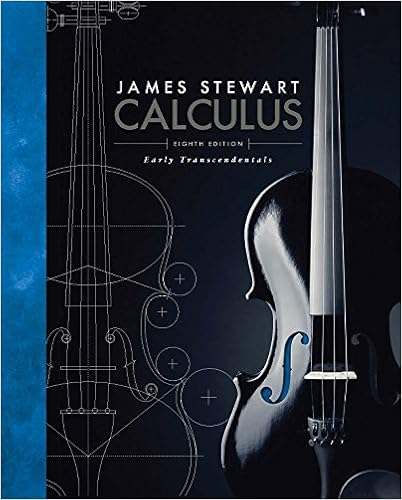# UFCalcSet5 - Exercises UF Calculus Set 5 1 Determine...

• Notes
• 3
• 100% (1) 1 out of 1 people found this document helpful

This preview shows page 1 - 2 out of 3 pages.

##### We have textbook solutions for you!
The document you are viewing contains questions related to this textbook.The document you are viewing contains questions related to this textbook.
Chapter 1 / Exercise 10
Calculus: Early Transcendentals
StewartExpert Verified
ExercisesUF Calculus Set 51. Determine whether the following represent one-to-one functions:(a) a rule that associates a person with his/her nuclear DNA(b) a rule that associates a person with his/her current set of fingerprints(c) a rule that associates, to a given time, the distance from a swinging pendulum to itslowest point(d) a rule that associates, to a given distance traveled, the speed of an object falling from abuilding without air resistence2. The functionf(x) =x+ex-1is one-to-one. By inspection, what isf-1(0)? what isf-1(e)?3. A tank continuously drains so that the volumeVof water (in gallons) remaining in the tankaftertminutes isV(t) = 100(1-t20)2.(a) What is the domain ofV(t)by the context of the problem? What is the range?(b) What is implied about the physical nature of the problem that ensuresV(t)is one-to-oneon its domain? Find the inverse function.(c) What isV-1(25)? Interpret its meaning in the given scenario.
##### We have textbook solutions for you!
The document you are viewing contains questions related to this textbook.The document you are viewing contains questions related to this textbook.
Chapter 1 / Exercise 10
Calculus: Early Transcendentals
StewartExpert Verified
•••